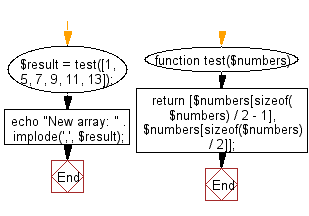﻿ PHP Exercises: Create an array taking two middle elements from a given array of integers of length even - w3resource# PHP Exercises: Create an array taking two middle elements from a given array of integers of length even

## PHP Basic Algorithm: Exercise-100 with Solution

Write a PHP program to create an array taking two middle elements from a given array of integers of length even.

Sample Solution:

PHP Code :

``````<?php
function test(\$numbers)
{
return [\$numbers[sizeof(\$numbers) / 2 - 1], \$numbers[sizeof(\$numbers) / 2]];
}

\$result = test([1, 5, 7, 9, 11, 13]);
echo "New array: " . implode(',', \$result);
``````

Sample Output:

```New array: 7 9
```

Flowchart:PHP Code Editor:

What is the difficulty level of this exercise?

﻿

## PHP: Tips of the Day

\$_REQUEST: This SuperGlobal Variable is used to collect data submitted by a HTML Form

Example:

This code save in a php file and run in the browser.

```<!DOCTYPE html>
<html>
<body>

<form method="post" action="<?php echo \$_SERVER['PHP_SELF'];?>">
NAME: <input type="text" name="user">
<button type="submit">SUBMIT</button>
</form>
<?php
if (\$_SERVER["REQUEST_METHOD"] == "POST") {
\$name = htmlspecialchars(\$_REQUEST['user']);
if(empty(\$name)){
echo "Name is empty";
} else {
echo \$name;
}
}
?>
</body>
</html>
```

Output:

```Owen
```